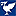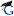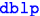# Economics and Computation Group

 Dr Michael Baneperformance-portable CPU|FPGA; energy efficient computing Dr. Giorgos ChristodoulouAlgorithmic game theory, inefficienty of equilibria, mechanism design, decision making under uncertainty Dr. John FearnleyAlgorithmic game theory, formal methods, logic and games Dr Aris Filos RatsikasAlgorithmic mechanism design without money; Social choice theory; Divisible and indivisible item allocation Dr. Martin GairingHead of GroupAlgorithmic game theory, efficiency of equilibria, combinatorial optimisation, approximation algorithms. the price of anarchy Dr. Rasmus Ibsen-jensenalgorithmic game theory; strategy complexity of two player, zero-sum games Prof. Piotr KrystaAlgorithmic game theory, approximation algorithms, combinatorial optimization, computational complexity, mechanism design Prof. Rida LarakiGame theory, social choice, economic theory, optimization, learning, and operations research Prof. Rahul SavaniComputational game theory, algorithms and complexity, equilibrium computation, automated and algorithmic trading Dr. Alkmini SgouritsaAlgorithmic game theory, mechanism design, algorithms Prof Paul SpirakisAlgorithms & complexity, algorithmic game theory, distributed algorithms, population protocols, temporal networks, theory of networks, sensor networks Dr. Konstantinos TsakalidisAlgorithm & Data Structures; Discrete & Computational Geometry; Graph Algorithms; Databases & Big Data Dr. Dominik WojtczakAlgorithmic game theory; Efficient analysis of probabilistic systems; Computer security; Algorithms and theory of automata
 Flavia Alves Machine Learning; Data Analysis; Game Theory; Optimisation Emmanouil Bakopoulos Nestoras Chalkidis Nikos ProtopapasAlgorithmic game theory, Algorithmic mechanism design Azhan Rashid Thomas SpoonerAutomated trading, Multi-agent Reinforcement Learning, Machine Learning